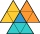# Expression of a variable from the formula + The Law of Sines - math problems

#### Number of problems found: 9

• Calculate triangleIn the triangle ABC, calculate the sizes of all heights, angles, perimeters and its area, if given a-40cm, b-57cm, c-59cm
• Triangle's centroidIn the triangle ABC the given lengths of its medians tc = 9, ta = 6. Let T be the intersection of the medians (triangle's centroid) and point is S the center of the side BC. The magnitude of the CTS angle is 60°. Calculate the length of the BC side to 2 d
• Viewing angleThe observer sees a straight fence 60 m long at a viewing angle of 30°. It is 102 m away from one end of the enclosure. How far is the observer from the other end of the enclosure?
• The aspect ratioThe aspect ratio of the rectangular triangle is 13: 12: 5. Calculate the internal angles of the triangle.
• Children playgroundThe playground has a trapezoid shape, and the parallel sides have a length of 36 m and 21 m. The remaining two sides are 14 m long and 16 m long. Find the size of the inner trapezoid angles.
• SSA and geometryThe distance between the points P and Q was 356 m measured in the terrain. The PQ line can be seen from the viewer at a viewing angle of 107° 22 '. The observer's distance from P is 271 m. Determine the viewing angle of P and observer.
• Largest angle of the triangleCalculate the largest angle of the triangle whose sides have the sizes: 2a, 3/2a, 3a
• Water channelThe cross section of the water channel is a trapezoid. The width of the bottom is 19.7 m, the water surface width is 28.5 m, the side walls have a slope of 67°30' and 61°15'. Calculate how much water flows through the channel in 5 minutes if the water flo
• Diamond diagonalsCalculate the diamond's diagonal lengths if its content is 156 cm2 and the side length is 13 cm.

We apologize, but in this category are not a lot of examples.
Do you have an exciting math question or word problem that you can't solve? Ask a question or post a math problem, and we can try to solve it.

We will send a solution to your e-mail address. Solved examples are also published here. Please enter the e-mail correctly and check whether you don't have a full mailbox.

Expression of a variable from the formula - math problems. The Law of Sines - math problems.